# Using DATE function in Excel - formula examples to calculate dates

When it comes to calculating dates in Excel, DATE is the most essential function to understand. As you probably know, Excel does not keep the year, month and day for a date, nor does it explicitly store weekday information in a cell. Instead, Microsoft Excel stores dates as serial numbers and this is the main source of confusion.

Not all Excel date functions can recognize dates entered as text values, therefore it's not recommended to supply dates directly in calculations. Instead, you should use the DATE function to get a serial number representing the date, the number that Excel understands and can operate on.

## Excel DATE function

The Excel DATE function returns the serial number that represents a certain date. It has the following arguments:

DATE(year, month, day)

Where:

Year - represents the year of the date.

Month - an integer representing the month of the year, from 1 (January) to 12 (December).

Day - an integer corresponding to the day of the month, from 1 to 31.

The DATE function is available in all versions of Excel 365 - Excel 2007.

### DATE function - tips and notes

The DATE syntax looks crystal clear and straightforward on the surface. In practice, there may be some unobvious pitfalls that the below tips will help you avoid.

#### Year

Excel interprets the year argument according to the date system set up on your computer. By default, Microsoft Excel for Windows uses the 1900 system where January 1, 1900 is represented by the serial number 1. For more details, please see Excel date format.

• If the year argument is between 1900 and 9999 inclusive, Excel uses exactly the value you supplied to create a date. For example, DATE(2015, 12, 31) returns December 31, 2015.
• If the year argument is between 0 and 1899 inclusive, Excel adds the specified number to 1900. For example, DATE(100, 12, 31) returns December 31, 2000 (1900 + 100).
• If the year argument is less than 0 or greater than 9999, a DATE formula will return the #NUM! error.

Tip. To avoid confusion, always supply four-digit years. For example, if you input "01" or "1" in the year argument, your DATE formula will return the year of 1901.

#### Month

• If the month argument is greater than 12, Excel adds that number to the first month in the specified year. For example, DATE(2015, 15, 5) returns the serial number representing March 1, 2016 (January 5, 2015 plus 15 months).
• If the month argument is less than 1 (zero or negative value), Excel subtracts the magnitude of that number of months, plus 1, from the first month in the specified year. For example, DATE(2015, -5, 1) returns the serial number representing July 1, 2014 (January 1, 2015 minus 6 months).

#### Day

As well as month, the day argument can be supplied as a positive and negative number, and Excel calculates its value based on the same principles as described above.

Tip. At first sight, supplying negative values in the month or day argument of the Excel DATE function may seem absurd, but in practice it may turn out quite useful, for example in the complex formula that converts a week number to a date.

## Excel DATE formula examples

Below you will find a few examples of using DATE formulas in Excel beginning with the simplest ones.

### Example 1. DATE formula to return a serial number for a date

This is the most obvious use of the DATE function in Excel.

For example, to return a serial number corresponding to 20-May-2015, use this formula:

`=DATE(2015, 5, 20)`

Instead of specifying the values representing the year, month and day directly in a formula, you can have some or all arguments driven by of other Excel date functions. For instance, combine the YEAR and TODAY to get a serial number for the first day of the current year.

`=DATE(YEAR(TODAY()), 1, 1)`

And this formula outputs a serial number for the first day of the current month in the current year:

`=DATE(YEAR(TODAY()), MONTH(TODAY(), 1)`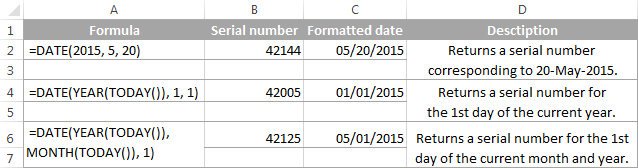Tip. To display a date rather than a serial number, apply the desired Date format to the formula cell.

### Example 2. How to return a date based on values in other cells

The DATE function is very helpful for calculating dates where the year, month, and day values are stored in other cells.

For example, to find the serial number for the date, taking the values in cells A2, A3 and A4 as the year, month and day arguments, respectively, the formula is:

`=DATE(A2, A3, A4)`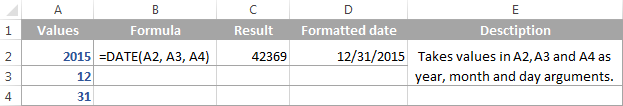### Example 3. DATE formula to convert a string or number to a date

Another scenario when the Excel DATE function proves useful is when the dates are stored in the format that Microsoft Excel does not recognize, for instance DDMMYYYY. In this case, you can use DATE in liaison with other functions to convert a date stored as a numeric string or number into a date:

`=DATE(RIGHT(A2,4), MID(A2,3,2), LEFT(A2,2))`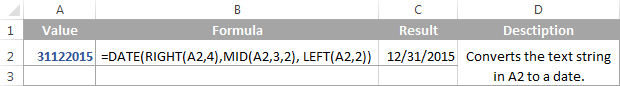### Example 4. Adding and subtracting dates in Excel

As already mentioned, Microsoft Excel stores dates as serial numbers and operates on those numbers in formulas and calculations. That is why when you want to add or subtract some days to/from a given date, you need to convert that date to a serial number first by using the Excel DATE function. For example:

• Adding days to a date:`=DATE(2015, 5, 20) + 15`The formula adds 15 days to May 20, 2015 and returns June 4, 2015.
• Subtracting days from a date:`=DATE(2015, 5, 20) - 15`The result of the above formula is May 5, 2015, which is May 20, 2015 minus 15 days.
• To subtract a date from today's date:`=TODAY()-DATE(2015,5,20)`The formula calculates how many days are between the current date and some other date that you specify.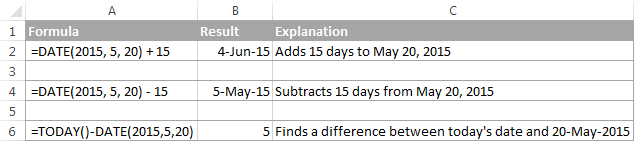If you are adding or subtracting two dates that are stored in some cells, then the formula is as simple as =A1+B1 or A1-B1, respectively.

And here are a few more examples where Excel DATE is used in combination with other functions in more complex formulas:

## Using Excel DATE formulas in conditional formatting

In case you want not only to calculate but also highlight dates in your Excel worksheets, then create conditional formatting rules based on DATE formulas.

Supposing you have a list of dates in column A and you want to shade dates that occurred earlier than 1-May-2015 in orange and those that occur after 31-May-2015 in green.

The DATE formulas you want are as follows:

Orange: `=\$A2<DATE(2015, 5, 1)` - highlights dates less than 1-May-2015

Green: `=\$A2>DATE(2015, 5, 31)` - highlights dates greater than 31-May-2015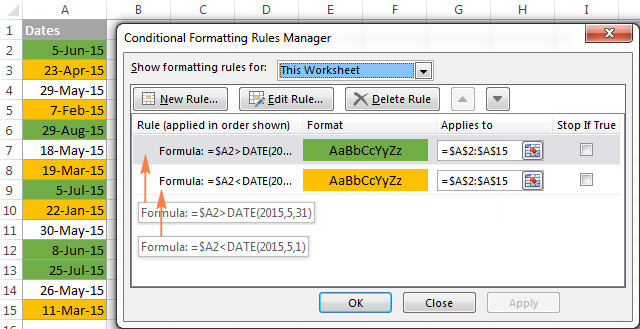For the detailed steps and more formula examples, please see How to conditionally format dates in Excel.

## How to make date formulas in Excel with Date & Time Wizard

Though DATE is the main function to work with dates in Excel, a handful of other functions are available to tackle more specific tasks. You can find the links to in-depth tutorials at the end of this article.

Meanwhile, I'd like to present you our Date & Time Wizard - a quick and easy way to calculate dates in Excel. The beauty of this tool is that outputs the results as formulas, not values. Thus you have a kind of 'two birds, one stone' opportunity - get the result faster and learn Excel date functions along the way :)

The wizard can perform the following calculations:

• Add years, months, weeks, days, hours, minutes and seconds to the specified date.
• Subtract years, months, weeks, days, hours, minutes and seconds from the specified date.
• Calculate the difference between two dates.
• Get age from the birthdate.

For example, here's how you can add 4 different units in cells B3:E3 to the date in A4. The formula in B4 is built in real-time as you change the conditions: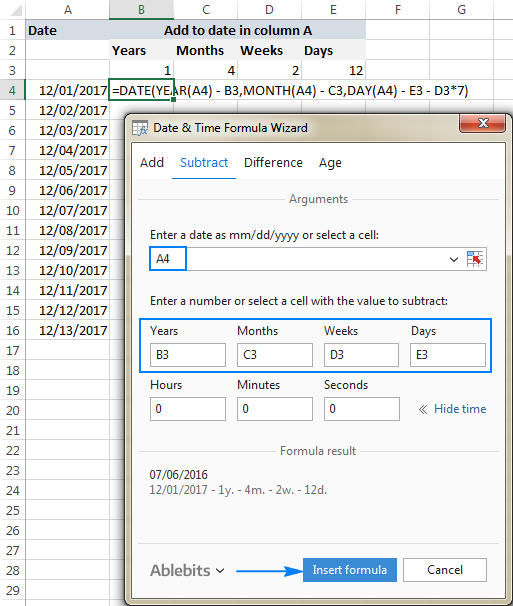If you are curious to explore other capabilities of the wizard, feel free to download an evaluation version of the Ultimate Suite below which includes this as well as 60 more time saving add-ins for Excel.

I thank you for reading and hope to see you on our blog next week!

## Excel dates tutorials:

1. When I deduct 2 dates from one another that are exactly 24 hours apart, the answer is returned as zero, not as 24 hours

2. Hello, please create a formula. I need to get the number of days overdue from the requested delivery date which automatically updates everyday.

Ex. Today is October 23rd, customer's requested delivery date is on the 20th. So the answer must be 3 days.

3. Date CELL Repeated Count Ticket#
02-Sep-23 3000092822 2 Ticket#102922098301
07-Sep-23 3000092822 2 Ticket#102698554587

ho can I find difference of dates in days from date column in an other column as I have same values in cell column

• Hi! If I understand your task correctly, the following formula should work for you:

=INDEX(A2:A10,MATCH(B1,B2:B10,0))-A1

Find the date in column A that has the same value in column B using INDEX MATCH function. Then find the date difference.

4. Dear Sir

Once again thank you so much.
My meaning is how to supply the start date directly to the the formula, use the DATE function

Submission date Sep/2023- Receiving comments Oct/2023- Last Rev. No. Good
Thank you

5. Dear Sir

My meaning is how to use with date.

• Hi! Unfortunately, this information is not enough to understand what you need. You can see how to use the IF function with a date in these instructions.

6. Hello, I need help..

But I'm not quite sure how to describe it. I wants to count the total numbers of working days based on days with figure > 1.

Eg. Work day is Mon to Sat. And certain days, the fig 1-100. So instead of counting total numbers, I wants to count it as total working days.

Mon = 20
Tues = 40
Wed = 0
Thurs = 60
Fri = 0
Sat = 0

So instead of 120, I wants to count it as 3 working days. How do I go about it?

• Hi! To count the number of values greater than 0, use the COUNTIF function. For example:

=COUNTIF(B1:B10,">0")

7. Dear Sir

how to fix this formulas if i have 3 column Ro0.Ro1.Ro2
if i will type date a cell that come to auto come Ro0 or Ro1 0r Ro2 or and i want just type date and come to

=IF(F8,G8.H8<=TODAY, "RO0","RO1", "RO2"))
Thank you

• Sorry, it's not quite clear what you are trying to achieve.

8. Hello, I created an inventory sheet. I have the count of each item in colum b and my goal is to associate a date in colum d when i enter a value in colum b. Is this even possible

9. I HAVE VALUES :
TYPE Name Date Amount Date2 Amount3
Pre ABDUL 01-03-2024 2000 03-03-2024 2001
post KAREEM 04-04-2024 4000 05-04-2024 4001
post Sharief 03-07-2023 3000 04-07-2023 3001

I need to enter the date specific in return i need amount of that month how can I enter formula ? to fetch the current month amount

10. what is the formula of getting the target completion date from the date received plus the SLA/TAT.

• I am not sure I fully understand what you mean.

11. How to compute the due date excluding weekends and holidays

12. If i'm monitoring runoff on a porfolio and each month I go in and change payments made, the fomula I have automatically decreases the payments remaining. However the next column is "next due" date. What formula would I use to advance the date one month ahead when the "payments remaining" column automatically decreases?

• Hi! Unfortunately, this information is not enough to recommend a formula to you. Describe your task in more detail. Give an example of the source data and the expected result.

• Hi. The spreadsheet has a loan portfolio. It contains the original amount, the monthly payment, the # of payments made, the # of payments remaining, date of next payment due and the outstanding balance. I go in and update the # of payments made and everyother cell recalculates the data except the next payment due date. I don't know how to formulate the date column to change to the next month (the day stays the same) when the # of paymetns remaining automatically subtracts.
cost payment term payments made remaining next due contract balance
\$60.00 \$1.00 60 48 12 09/20/2023 \$12.00

• Hi. I’m sorry, but your description doesn’t give me a complete understanding of your task. Maybe this guide will be helpful: Add months to date in Excel.

13. what is the formula to get a result of 20th from a cell containing a August 20, 2023. thanks

14. I have a date column that auto populates 30 days from a previous colun usign a sum function. I am trying to figure out or nest the workday function into this column so if the 30 day date falls on a sat or sun it would revert to the previous Friday date

• Hi! A nested IF function including WEEKDAY should be used to check the day of the week:

=IF(WEEKDAY(A2+30)=1,(A2+30)-2,IF(WEEKDAY(A2+30)=7,(A2+30)-1,A2+30))

15. i have a date (mmm-yy) in Column A, Column D represents a number (1, 2 or 3) - I wish to write a formula for if column A shows a month/year, and column D shows number 1, new column should show a date 3 months into future.
Number 1: 1 month into future
Number 2: 3 months into future
Number 3: 6 months into future
Eg.
Date: Jun-23
Number: 1
New Date: Jul-23

Date Jun-23
Number: 2
New Date: Sep-23

Date: Jun-23
Number: 3
New Date: Dec-23

Thanks!

16. Is it possible to combine dates in text strings to not repeat days, months, or years if both dates have them in common?

Example:
"15 Aug 23-15 Aug 23" would be "15 Aug 23" (day/month/year not repeated)
"15 Aug 23-16 Aug 23" would be "15-16 Aug 23" (month/year not repeated)
"15 Aug 23-16 Sep 23" would be "15 Aug-16 Sep 23" (year not repeated)
"15 Aug 23-16 Jan 24" would be "15 Aug 23-16 Jan 24" (my formula renders this correctly as day/month/year are different)

Here's the formula I'm currently using to get the first renderings:
=TEXT(A1,"d mmm yy")&"-"&TEXT(B1,"d mmm yy")
A1: Start Date
B1: End Date

Is this possible? I don't think my current formula is actually on the right path. Is there a way to make Excel return a date range (15-16 Aug 23) given a start date and number of days long (15 Aug 23, 2) or something? Since I have start and end dates, I can calculate length in days in a third column and then use that data in the correct formula in column 4.

Thanks!

• Okay, so I was able to figure out a work around using IF() formulas after breaking out Month and Year in their own columns to compare against.

A1: Start Date
B1: End Date
C1: Start Month
D1: End Month
E1: Start Year
F1: End Year
G1: =IF(D1>C1,TEXT(A1,"d mmm"&"-"),IF(A1=B1,"",TEXT(A1,"d"&"-")))&TEXT(B1,"d mmm yy")

I'm sure there's a cleaner way, but this produces the outcome I want. I realized that the end date is always used, it's the start date that is modified depending on it's commonality to the end date. A one day event only needs to show the end date. An event spanning several days of the same month only needs the days from the start date. An event spanning two months needs the day and month from the start date but not the year. An event spanning two years needs the entire start date with the end date.

This is probably the most complex thing I've done in Excel. I welcome any feedback!

• Sorry, copy/paste issues, here is the right formula text string, I also put the logic questions in a logical order:

A1: Start Date
B1: End Date
C1: Event Name
D1: Start Month C1
E1: End Month D1
F1: Start Year E1
G1: End Year F1
F1 =(IF(A1=B1,(TEXT(B1,"d mmm yy")),(IF(E1=D1,(TEXT(A1,"d")&"-"&TEXT(B1, "d mmm yy")),IF(F1=G1,(TEXT(A1,"d mmm")&"-"&TEXT(B1, "d mmm yy")),(TEXT(A1,"d mmm yy")&"-"&TEXT(B1,"d mmm yy"))))))&": "&C1)

17. Hi,

I am looking to create a formula that will take the date from one cell (say it is cell B2, data is 08/02/2023) and in a new cell, have an IF statement that would produce the following:

If the date in cell B2 is less than the date in G2 (which is currently a formula of if(isblank(B2),"",B2+30) the new cell would be labeled as "On Hold" but if the date in B2 is greater than the date in G2 the new cell would be labeled as "Past Due".

This would need to be two arguments, but I am killing myself trying to figure it out and haven't been able to find the correct way to write the formula.

Any help would be appreciated.

Thank you!

18. I want to convert 11.50 to years months and days, ex: 11.50 is 11 years 6 months and 0days

• Hi! If I understand your task correctly, the following formula should work for you:

=INT(A2)&" years "&INT((A2-INT(A2))*12)&" months "&INT(((A2-INT(A2))*10-INT((A2-INT(A2))*10))*30)&" days"

19. I am trying to create a clock calendar counter in excel.

if i have one column start date 7/1/203 the next column want an auto set counter to count 21 days from prior date then next column to count 30 days from previous date and last count 90 days from previous date

Thank you

20. how to sum total in excel output same format in excel
1 years, 9 months, 26 days
1 years, 8 months, 25 days
0 years, 3 months, 11 days
0 years, 5 months, 0 days

• Hi! I assume your data is text. Therefore you cannot summarize them.

21. I am trying to set a formula for days counter
To subtract a date from today's date: =TODAY()-DATE(2015,5,20) The formula calculates how many days are between the current date and some other ...
I use a date entered in separate column -- say "H"
so result "L" = (TODAY()-(Hxx)) where xx is the line-number
but I need to use a condition as follows:
if value in column "K" is null or non-numeric (as text), then "L" = (TODAY()-(Hxx)), otherwise "L" = (TODAY()-(Kxx))
i.e. I need to use a date from column "H" or column "K", depending on - whether the date is entered in column "K". But if column "K" is blank or has some notes (optional), then the conditional formula should use value of the column "H".
Could anyone suggest that type of conditional formula?
Thank you

• Great, Alexander !
Thank you very much !! it works well.

22. December, 21 2022 Date Change Formate 21/12/2022

23. Hello, I need your help to get a formula to calculate below,

below are the dates and holidays. Please recreate the formula to get exact working hours on monthly.

Conditions : -

23 March to 1o April - 6 working hours per day
11 April to 19 April - 5 working hours per day
23 march to april 19 - 3 working hours on friday
Rest of the working days - 8 working hours and 6 working hours on friday

Date Holidays
January 20-Apr-23
February21-Apr-23
March 27-Jun-23
April 28-Jun-23
May 29-Jun-23
June 30-Jun-23
July 01-Dec-23
August
September
October
November
December

24. Can you correct a date where the month was incorrectly entered as a year??
For example:
Jan 1, 2023 was entered as '2001-1-23'

How can I correct it to read '01/01/23'

• Split this value into text strings with "-" delimiters using the TEXTSPLIT function. Use these in the DATE function to get the date.